Next: Comparison of Indexing and Up: Indexing & Hashing Previous: Hash Indices

# Dynamic Hashing

1. As the database grows over time, we have three options:
• Choose hash function based on current file size. Get performance degradation as file grows.
• Choose hash function based on anticipated file size. Space is wasted initially.

• Periodically re-organize hash structure as file grows. Requires selecting new hash function, recomputing all addresses and generating new bucket assignments. Costly, and shuts down database.
2. Some hashing techniques allow the hash function to be modified dynamically to accommodate the growth or shrinking of the database. These are called dynamic hash functions.
• Extendable hashing is one form of dynamic hashing.
• Extendable hashing splits and coalesces buckets as database size changes.
• This imposes some performance overhead, but space efficiency is maintained.
• As reorganization is on one bucket at a time, overhead is acceptably low.
3. How does it work?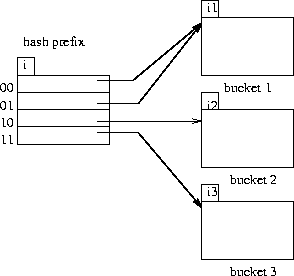Figure 11.9:   General extendable hash structure.

• We choose a hash function that is uniform and random that generates values over a relatively large range.
• Range is b-bit binary integers (typically b=32).
•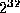is over 4 billion, so we don't generate that many buckets!
• Instead we create buckets on demand, and do not use all b bits of the hash initially.
• At any point we use i bits where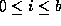.
• The i bits are used as an offset into a table of bucket addresses.
• Value of i grows and shrinks with the database.
• Figure 11.19 shows an extendable hash structure.
• Note that the i appearing over the bucket address table tells how many bits are required to determine the correct bucket.
• It may be the case that several entries point to the same bucket.
• All such entries will have a common hash prefix, but the length of this prefix may be less than i.
• So we give each bucket an integer giving the length of the common hash prefix.
• This is shown in Figure 11.9 (textbook 11.19) as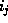.
• Number of bucket entries pointing to bucket j is then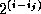.
4. To find the bucket containing search key value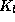:
• Compute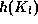.
• Take the first i high order bits of.
• Look at the corresponding table entry for this i-bit string.
• Follow the bucket pointer in the table entry.
5. We now look at insertions in an extendable hashing scheme.
• Follow the same procedure for lookup, ending up in some bucket j.
• If there is room in the bucket, insert information and insert record in the file.
• If the bucket is full, we must split the bucket, and redistribute the records.
• If bucket is split we may need to increase the number of bits we use in the hash.
6. Two cases exist:

1. If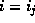, then only one entry in the bucket address table points to bucket j.

• Then we need to increase the size of the bucket address table so that we can include pointers to the two buckets that result from splitting bucket j.
• We increment i by one, thus considering more of the hash, and doubling the size of the bucket address table.
• Each entry is replaced by two entries, each containing original value.
• Now two entries in bucket address table point to bucket j.
• We allocate a new bucket z, and set the second pointer to point to z.
• Setand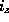to i.
• Rehash all records in bucket j which are put in either j or z.
• Now insert new record.
• It is remotely possible, but unlikely, that the new hash will still put all of the records in one bucket.
• If so, split again and increment i again.
2. If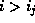, then more than one entry in the bucket address table points to bucket j.
• Then we can split bucket j without increasing the size of the bucket address table (why?).
• Note that all entries that point to bucket j correspond to hash prefixes that have the same value on the leftmostbits.
• We allocate a new bucket z, and setandto the originalvalue plus 1.
• Now adjust entries in the bucket address table that previously pointed to bucket j.
• Leave the first half pointing to bucket j, and make the rest point to bucket z.
• Rehash each record in bucket j as before.
• Reattempt new insert.
7. Note that in both cases we only need to rehash records in bucket j.
8. Deletion of records is similar. Buckets may have to be coalesced, and bucket address table may have to be halved.
9. Insertion is illustrated for the example deposit file of Figure 11.20.
• 32-bit hash values on bname are shown in Figure 11.21.
• An initial empty hash structure is shown in Figure 11.22.
• We insert records one by one.
• We (unrealistically) assume that a bucket can only hold 2 records, in order to illustrate both situations described.
• As we insert the Perryridge and Round Hill records, this first bucket becomes full.
• When we insert the next record (Downtown), we must split the bucket.
• Since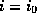, we need to increase the number of bits we use from the hash.
• We now use 1 bit, allowing us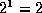buckets.
• This makes us double the size of the bucket address table to two entries.
• We split the bucket, placing the records whose search key hash begins with 1 in the new bucket, and those with a 0 in the old bucket (Figure 11.23).
• Next we attempt to insert the Redwood record, and find it hashes to 1.
• That bucket is full, and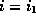.
• So we must split that bucket, increasing the number of bits we must use to 2.
• This necessitates doubling the bucket address table again to four entries (Figure 11.24).
• We rehash the entries in the old bucket.
• We continue on for the deposit records of Figure 11.20, obtaining the extendable hash structure of Figure 11.25.